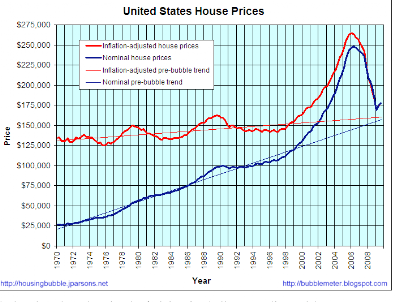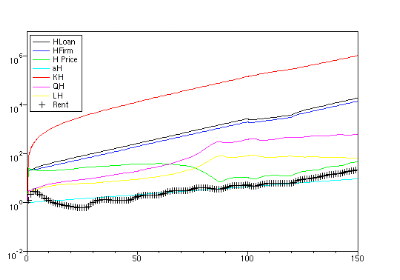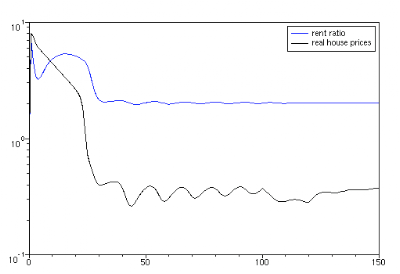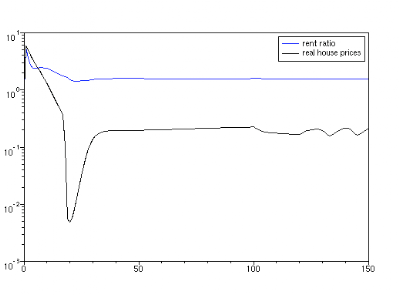Thursday, March 18, 2010

Rental vacancies and house prices

The first figure below is from a simulation of a toy economy I ran based on Steve Keen's model.I detected an obvious relationship between rental vacancies and house prices. The rental vacancy peaks ahead of the house price peak and occurs at about midpoint between the house price bottoms and tops. After the rental vacancy peaks, the house prices increase until the rental vacancy reaches a low level. The rental vacancy approaching a bottom is an indicator of a house price top.

I wondered if this relationship held up in the real world. The second and third figures show US house prices and rental vacancies.The house price shows a bottom in 1976 and a top in 1979. The rental vacancy peaks at 6+% in 1975 and declines to 5% in 1978. The rental top and bottom anticipated the house price movement.

The house price then declined from 1979 to 1983. The rental vacancy then blipped up a bit from 1979 to 1981 and then declined to a relative bottom in 1981-82. Again, the rental vacancy anticipated the house prices.

Beginning in 1982, the rental vacancy rose steadily from 5% to a peak in 1988 of 8%. The rental vacancy then declined from 1988 to 1990 to another relative bottom. The house prices during this time bottomed in 1983 and rose to a top in 1990. The peak rental vacancy anticipated the price top by 2 years.

The rental vacancy bottomed at slightly under 7% in 1994 and then rose gradually until 2001. The house price during this time bottomed in 1996-97 and then climbed steeply to a top in 2006. The top of the house price rise was anticipated by the rental vacancy peak of 10+% of 2004. The relative bottom of rental vacancy was simultaneous with the price top in 2006.

I conclude that the rental vacancy-house price relationship predicted by the simulation is substantiated by the empirical data.

The program code is attached for interested SciLabbers.

z0=[500;500;0;0;1;1;1;1000;0;0;0;500;20;20;1;1;80;10;10;1;100];
t=0:1:200;
clf()

function dx = keeninterm(t,x)

rL=.05
rD=.03
s=.3

tau_HQ=1

v=5
HV=26/4

sH=0.3

tauS=1
tauW=1/(26-HV)

tau_Q=1;tau_L=1
tau_H=1

tau_pi=1,tau_piH=1

tausH=8;tauWH=1/HV;

//if t> 160 then
//fac=.95;
//else fac=1.0;
//end
//if t>190 then
//fac=1.0
//end
fac=1

tauL=7*fac
tauR=1/fac
tauM=16/fac
tauMH=16/fac

tauB=1.25;tauBH=5
tauP=1

alpha=.015;alphaH=.015;
bta=.02

gma=.01;gmaH=.01

stimH=0
//if t>75 then
//stimH=.4
//end

A=rL*x(1); B=A; C=rD*x(2);D=(1-s)/tauS*x(2);E=rD*x(3);F=x(3)/tauW;G=x(4)/tauB;
H=x(1)/tauL;
I=x(9)*x(2)/((x(2)+x(14))*tauR);
J=x(2)/tauM;

HA=rL*x(13);HB=HA;HC=rD*x(14);HD=(1-sH)/tausH*x(14);HF=x(3)/tauWH;HH=x(13)/tauL;HI=x(9)*x(14)/((x(2)+x(14))*tauR);HJ=x(14)/tauMH
HG=x(4)/tauBH;LT=x(12)+x(19);c=x(18)/LT;vacancy=x(21)-(LT-x(18))

//loss=x(20)*vacancy*x(21)/(x(21)-vacancy)
loss=0
HK=x(17)-loss

CF=1-2*c+stimH

SS=c*(1-c)*LT^2
SSmax=.25*LT^2

if (c^2)^.5 <1 ssrat="SS/SSmax" ssrat="1" ge="genexp(x,xv,yv,s,m)" ge="(yv-m)*exp(s/(yv-m)*(x-xv))" lamda="(x(12)+x(19))./x(8)" ph="genexp(lamda,.97,0,1.5,-.06)

unemp=1-lamda

dx(1)=A-B-H+I+J
dx(2)=-B+C-D+F+G-H+I+J
dx(3)=D+E-F+HD-HF
dx(4)=B-C-E-G+HB-HC-HG
dx(5)=-1/tauP*(x(5)-x(6)./((1-s)*x(7)))
dx(6)=Ph*x(6)
dx(7)=alpha*x(7)
dx(8)=bta*x(8)
dx(9)=H-I-HI+HH

dx(10)=x(2)/tau_pi-gma*x(10)
dx(10)=x(2)/tauS-gma*x(10)

dx(11)=-.25/tau_Q*(x(11)-x(10)/(v*x(5)))
dx(12)=-1/tau_L*(x(12)-x(11)/x(7))

Profit=x(5)*x(11)-x(6)*x(12)-A+C
ProfitH=x(15)*x(18)-x(6)*x(19)-HA+HC

k=SSrat

k_H=1

dx(13)=HA-HB-HH+HI+HJ

cbar=.10*(.3*x(6)+.7*x(5));
Cbar=.15*(.7*x(6)+.3*x(5));

//ProfitH=HProfit+RProfit

dx(14)=-HB+HC-HD+HF+HG-HH+HI+HJ//+Profit

//HP=HK*(1+.5*exp(-((t-107)/10)^2)-.2*exp(-((t-120)/10)^2))
HP=HK*(1+.5*exp(-((t-107)/10)^2))//-.2*exp(-((t-120)/10)^2))
//HP=HK
//if t>108 then
//HP=HK*(1+.25*exp(-((t-107)/10)^2)-.5*exp(-((t-112)/10)^2))
//end

dx(15)=-k/tauP*(x(15)-(HP-x(20)*x(21))/(10*x(18)))

dx(16)=x(16)*alphaH

dx(17)=x(14)/tausH-gma*x(17)

dx(18)=-CF/tau_HQ*(x(18)-(HK*(1-sH)-cbar*x(21))/Cbar)

dx(19)=-.25/tau_L*(x(19)-(.7*x(18)+.3*x(21))/x(16))

dx(20)=-1/tauP*(x(20)-(HK-x(15)*x(18))/(10*x(21)))

//dx(21)=-.1/tau_HQ*(x(21)+vacancy-(HK*(1-sH)-Cbar*x(18))/cbar)
dx(21)=-.1/tau_HQ*(x(21)-1.3*(LT-x(18)))
//Pratio=x(15)/x(20)

//Cratio=Cbar*x(18)/(cbar*x(21))

endfunction

z=ode(z0,0,t,keeninterm);

LT=z(19,:)+z(12,:);
c=z(18,:)./LT;
Profit=z(5,:).*z(11,:)-z(6,:).*z(12,:)+.03*z(2,:)-.05*z(1,:);
lamda=(z(12,:)+z(19,:))./z(8,:);

vacancyrate=(z(21,:)-(LT-z(18,:)))./z(21,:);

unemp=1-lamda;
u=z(1:12,:);
uu=z(13:21,:);
plot2d(t',u',[1 2 3 4 5 6 7 -1 -2 -3 -4 -5 ],logflag="nl",rect=[0,.01,300,10^8]);
legends(['LoanF';'DepositF';'WD';'BD';'Price';'Wages';'a=prod.';'N';'BReserves';'K';'Q';'L'],[1 2 3 4 5 6 7 -1 -2 -3 -4 -5],"ur");
halt
plot2d(t',[Profit;unemp]',logflag="nl");
legends(['Profit';'Unemployment'],[1 2],"ul");
halt
clf()
plot2d(t',[uu; unemp]',[1 2 3 4 5 6 7 -1 -2 1],logflag="nl",rect=[0,.01,300,10^7])
legends(['HLoan';'HFirm';'H Price';'aH';'KH';'H';'LH';'rent';'R';'unemp'],[1 2 3 4 5 6 7 -1 -2 1],"ur");
halt
rentratio=z(15,:)./z(20,:);
clf()
plot2d(t',[z(15,:)./z(5,:);c;z(20,:)./z(5,:);unemp;vacancyrate]',style=[1 5 4 6 2],logflag="nl",rect=[0,.01,300,100])
legends(['real house prices';'c=H/LT';'real rent';'unemployment';'vacancy rate'],[1 5 4 6 2],"ur");
halt
clf()

plot2d4(c',[z(15,:)./z(5,:);rentratio]',[1 2])//,leg="real house prices vs c=H/L");
legends(['real house prices vs c=H/L';'rent ratio'],[1 2],"ur");
halt

Thursday, February 25, 2010

Simulation of Reduced Housing Supply

THE EFFECT OF REDUCED HOUSING SUPPLY

In previous simulations, I investigated the effects of manipulating house prices through pricing velocity. While that is , no doubt, a factor in maintaining high prices, here I study only the effect of keeping the supply of houses below the demand. In other words, restricting the supply to the higher end of the market.

High pricing velocity requires the supposition of unseen financial forces which can only be guessed at.

Reduced housing supply, on the other hand, only requires some reflection on the best interests of the housing industry.

Interests of the Housing Industry

The housing industry is, after all, the original housing speculator. In the interest of profitablity, the housing industry seeks to maintain the period of high prices for as long as possible. It is always more profitable to aim to build the highest priced housing possible. It is not in the builder's interest for the market to reach equilibrium. Once equilibrium is reached, housing demand falls in line with the normal growth of the economy. Bubble profits are then gone.This figure shows the progression of the housing sector under normal circumstances. The housing price is in line with the supply. The first houses to be built are very expensive. (In a city nearby to me, the oldest houses in town are mansions built by lumber barons during the lumber boom of the 1870s)

Prices gradually decline through the income strata of the population, and, with growing wages, the market reaches an equilibrium in about 30 years where half the employed population are home owners and the other half are renters.

The cost differential between owning a house and renting is shown by the difference between the green house price line and the hatched renter line.

At year 75, the government institutes a stimulus program to increase home ownership and make housing more affordable. This causes the house prices in the market to instantly decline. At the same time, the equilibrium home ownership percentage increases form 50% to 70%.

Remember, in an natural market D = 1 – 2*c , where c = H/LT , H = number of Houses,

LT = total number of employed households for the entire economy.

In a stimulated housing market, D = 1 – 2*c +stimH. In this study, stimH is assumed to be 0.4

The market is in equilibrium when D, the demand for new houses is zero. For this study, c = 0.7, meaning the market is in equilibrium when 70% of the employed households own houses, after market stimulation.This figure shows the rent ratio (the housing cost of owners/renters), along with the real house price

(house price/general wage level), and percentage home ownership c for the unmanipulated assumptions.

Next, I studied the effect of reducing the supply velocity of houses. I assumed that the housing industry only built housing that was most profitable, ie larger houses for the higher end of the market.

The housing supply velocity is given by the formula k*CF/tau_HQ. Here, tau_HQ is the supply lag time, CF is the demand metric = 1-2*c + stimH, and k is the supply velocity coefficient.

In the previous case, k = 1 throughout.

For the case shown below, I assumed for 0 < k ="1" k =" 0.25*(1+">

where k declines linearly from a little less than 0.5 to 0.25. After 60 years, k is constant at 0.25This figure shows the effect on the housing sector of reduced housing supply. The housing boom, the period of high prices, is considerably extended. The market comes into equilibrium at about t = 70. This is followed by a housing stimulus at t = 75 and the house price level is then brought down to a low multiple of the rents. At this point, demand is then very low and the effect of supply restriction unimportant.

Under the reducing supply regime, real house prices decline much more gradually. The housing stimulus at t = 75 greatly reduces real house prices as well. The credit crunch for 100 <>

The home ownership percentage grows to 70% at about t = 85I include an annotated SciLab code

z0=[100;100;0;0;1;1;1;300;0;0;0;100;20;20;1;1;1;1;2;1];

t=0:1:150;

clf()

function dx = keeninterm(t,x)

rL=.05

rD=.03

s=.3

v=5;

HV=5.2

tauS=.25

tauW=1/(26-HV)

tau_Q=1;tau_L=1

tau_H=1

tau_pi=1,tau_piH=1

tau_HQ=1;tau_HL=1;

sH=0.3;tausH=.25;tauWH=1/HV;

if t> 100 then

fac=.5;

else fac=1;

end

if t>120 then

fac=1

end

tauL=7*fac

tauR=1/fac

tauM=20/fac

tauMH=20/fac

tauB=1.25;tauBH=5

tauP=1

alpha=.015;alphaH=.015;

bta=.01

gma=.01;gmaH=.01

stimH=0

if t > 75 then

stimH=0.4

end

//FL=x(1);FD=x(2);WD=x(3);BD=x(4);P=x(5);wages=x(6);a=x(7);N=x(8);BR=x(9);K=x(10);

//Q=x(11); L=x(12)

//HL=x(13); HD=x(14); PH=x(15); aH=x(16);KH=x(17); H=x(18); LH=x(19); R=x(20)

A=rL*x(1); B=A; C=rD*x(2);D=(1-s)/tauS*x(2);E=rD*x(3);F=x(3)/tauW;G=x(4)/tauB;

H=x(1)/tauL;

I=x(9)*x(2)/((x(2)+x(14))*tauR);

J=x(2)/tauM;

HA=rL*x(13);HB=HA;HC=rD*x(14);HD=(1-sH)/tausH*x(14);HF=x(3)/tauWH;HH=x(13)/tauL;HI=x(9)*x(14)/((x(2)+x(14))*tauR);HJ=x(14)/tauMH

HG=x(4)/tauBH;LT=x(12)+x(19);c=x(18)/LT;DH=HV*x(3);DHR=x(3)*HV;HK=x(14)/tausH

CF=1-2*c+stimH

function GE=genexp(x,xv,yv,s,m)

GE=(yv-m)*exp(s/(yv-m)*(x-xv))

endfunction

lamda=(x(12)+x(19))./x(8)

Ph=genexp(lamda,.96,0,1,-.04)

dx(1)=A-B-H+I+J// dFL/dt

dx(2)=-B+C-D+F+G-H+I+J// dFD/dt

dx(3)=D+E-F+HD-HF// dWD/dt

dx(4)=B-C-E-G+HB-HC-HG// dBD/dt

dx(5)=-1/tauP*(x(5)-x(6)./((1-s)*x(7)))// dP/dt general price level

dx(6)=Ph*x(6)// dw/dt general wage level

dx(7)=alpha*x(7)// da/dt productivity

dx(8)=bta*x(8)// dN/dt population

dx(9)=H-I-HI+HH// dBR/dt bank reserves

dx(10)=x(2)/tau_pi-gma*x(10) // dK/dt das Kapital

dx(11)=-1/tau_Q*(x(11)-x(10)/(v*x(5)))// dQ/dt real output

dx(12)=-1/tau_L*(x(12)-x(11)/x(7))// dL/dt number of employed workers (households)

Profit=x(5)*x(11)-x(6)*x(12)-A+C

ProfitH=x(15)*x(18)-x(6)*x(19)-HA+HC

dx(13)=HA-HB-HH+HI+HJ

dx(14)=-HB+HC-HD+HF+HG-HH+HI+HJ

k=1 // PH velocity coefficient

k_H=1// H quantity velocity coefficient

if t>10 then

k_H=0.25*(1+(60-t)/60)

end

if k_H<.25 then

k_H=.25

end

dx(15)=-k/tauP*(x(15)-HK*(1-c)/x(18)) // dPH/dt House prices

dx(16)=x(16)*alphaH// housing sector productivity

dx(17)=x(14)/tauS-gma*x(17)// dKH/dt Housing Capital

dx(18)=-k_H*CF/tau_HQ*(x(18)*exp(.691/70)-HK/(x(15)))// dH/dt Housing supply

dx(19)=-1/tau_L*(x(19)-x(18)/x(16))// dL/dt employed housing workers

dx(20)=-1/tauP*(x(20)-HK*(1-c)/LT)// dR/dt Rents

endfunction

z=ode(z0,0,t,keeninterm);

c=z(18,:)./(z(19,:)+z(12,:));

Profit=z(5,:).*z(11,:)-z(6,:).*z(12,:)+.03*z(2,:)-.05*z(1,:);

lamda=(z(12,:)+z(19,:))./z(8,:);

unemp=1-lamda;

u=z(1:12,:);

uu=z(13:20,:);

plot2d(t',u',[1 2 3 4 5 6 7 -1 -2 -3 -4 -5 ],logflag="nl",rect=[0,.01,150,10^7]);

legends(['LoanF';'DepositF';'WD';'BD';'Price';'Wages';'a=prod.';'N';'BReserves';'K';'Q';'L'],[1 2 3 4 5 6 7 -1 -2 -3 -4 -5]);

halt

plot2d(t',[Profit;unemp]',logflag="nl");

legends(['Profit';'Unemployment'],[1 2],"ur");

halt

clf()

plot2d(t',uu',[1 2 3 4 5 6 7 -1],logflag="nl",rect=[0,.01,150,10^7])

legends(['HLoan';'HFirm';'H Price';'aH';'KH';'QH';'LH';'Rent'],[1 2 3 4 5 6 7 -1],"ul");

halt

rentratio=z(15,:)./z(20,:);

clf()

plot2d(t',[rentratio;z(15,:)./z(6,:);c]',style=[2 1 5],logflag="nl")

legends(['rent ratio';'real house prices';'c=H/LT'],[2 1 5],"ur");

Tuesday, February 23, 2010

Juicing the Housing Market

JUICING THE HOUSING MARKET WITH HOUSE PRICING VELOCITY, part 1

I’ve been working with Steve Keen's multisector computer model. I have set up a simulation for a housing sector along with the rest of the economy, ie, a 2 sector model. Using it, I believe I have discovered a simple way to create a housing bubble by merely juicing the housing price velocity.

The figure shown represents housing demand for a ‘natural market’ ie no stimulus, as well as new housing demand for a stimulated market.Letting c = H/L, where c is the percentage of homes (and homeowners),H is the number of homes, L is the number of employed households. c is also the percentage of potential sellers, and 1-c is the percentage of potential house buyers. S= c*(1-c) is the number of possible sales transactions. S is a maximum at c = 0.5. D = dS/dc = 1-2c is the demand for new houses,
D > 0 for c < 0.5, more buyers than sellers. When c moves beyond 0.5 there is selling pressure, D < 0

For a stimulated market D = 1-2c+stimH. For stimH = 0.3, D = 1.3 -2c and demand is in equilibrium at c = 0.65. In a stimulated market like this, there are 65% potential sellers and 35% potential buyers at equilibrium, which is to say there is a built-in oversupply of houses.

JUICING THE HOUSING MARKET WITH HOUSE PRICING VELOCITY, part 2

Empirical backing for part 1 is seen in the figure, reproduced for the above link. In the US market before 1944, there was a natural market for housing. The curve shows that the market had stabilized a c = 0.47 or 47% before 1944. In 1944, returning GIs took advantage of a housing stimulus known as VA loans which helped them to buy affordable housing. This program increased homeownership to 65% by 1980 or so. After that various FHA stimulus increased c to as high as 69%JUICING THE HOUSING MARKET WITH HOUSE PRICING VELOCITY, part 3

I modified the single sector model with a parallel housing sector. To get housing prices different from the general price level I wrote

dPH/dt = -1/tauPH(PH-FDH/tauS*(1-c)/H)

where FDH is the housing sector account, (1-c) is the buyer's percentage, H the number of houses and 1/tauPH is the house pricing velocity;

dH/dt= -CF/tauQ(H*exp(.691/70)-(FDH/tauS)/PH)

where CF = (1-2c + stimH) is the demand metric explained in part 1, tauQ is the housing supply lag (inverse velocity). The exponential term is included for depreciation. I assumed that the half life of a house was 70 years.

dLH/dt= -1/tauLH(LH - H/aH) is the employed households in the housing sector. To get the entire number of employed households LH is added to L, the employed households in the rest of the economy, to give LH+L=LT. aH is the housing sector productivity, the number of housing units/ per worker year.

I also included rents for the housing sector

dR/dt = -1/tauP(R-FDH/tauS*(1-c)/LT)

dKH/dt=-1/tauP(FDH/tauS- .01*KH) is investment.

tauP = 1 throughout except for the pricing equation where I multiplied by 1, 3, or 5 to increase the pricing velocity for tauP= 1, 0.33 and 0.2 respectively.

I assumed that housing was 20% of worker consumption. I defined HV = 0.2*26 = 5.2. For the housing sector, consumption lag was 1/HV and for the rest of the economy 1/tauW was
1/(26-HV). This took care of intersectoral demand.

In running the simulation, I couldn't get much of a housing bubble for the normal tauPH=1. So, like any good number cruncher, I started fiddling with the parameters. I discovered that I got a good bubble by choosing tauPH = 0.33 and I got a really good bubble using tauPH = 0.2

In thinking of how to justify this fiddling, a light bulb came on. I realized that is how the banksters did it! The securitized mortgages was how they boosted pricing velocity. The local banks cleaned up their liabilities by passing the debts on to the pension funds so that they could keep writing liars loans to any and all comers! The sheer velocity of the lending drove the prices up.The first attachment, entitled tauPH=.33, stimH=0 shows a good bubble which lasts for about 90 years. The bubble comes to an end when c= 0.5, the equilibrium H/LT. The bubble lasts so long because house prices are so high. The second attachment,stimH=0 ,tauPH=0.33 shows the rent ratio and the real house prices, ie the house prices divided by the wage level.

After the housing bubble, the housing cost is mostly rents and a small amount of new housing and converges to about 0.25w, which was slightly above the initial assumption of 0.2w This is to be compared with the attachmentstimH=0.3, tauP=0.33 which shows that the effect of the stimulus is to depress both the rent ratio and the real house price.

The attachment tauP=0.33, stimH=0.3 when compared with its no-stimulus companion shows that the stimulus causes the bubble to be much shorter lived.JUICING THE HOUSING MARKET WITH HOUSE PRICING VELOCITY, part 4

The attachments in this part are to be compared with the attachments of part 3. This part shows the extreme limits of juicing through pricing velocity.tauP=0.2, stimH=0 is a natural market with a perpetual housing boom. The extreme pricing velocity makes the prices very high. The boom never ends because the equilibrium point of c=0.5 is never reached. Indeed, houses are owned by less than 1% of the employed households.stimH=0, tauP=0.2 shows the rent ratio and real house prices for the same assumptions. House prices are around 100 times rent and real house prices cost 40 years of wages.

Of course, all that is needed to maintain such a scenario is an infinite supply of pension funds to keep buying the colossal mortgages.tauP=0.2, stimH=0.3 shows that after about 65 years of high government boosting the housing boom can finally be brought to an end, which is to say, that after 65 years of committing the entire national budget to house buying that 65% of the employed households will own homes. The only problem is -tauP=0.2, stimH=0.3 - that after paying 20-25 years worth of real wages for a home during the boom, the homeowner will find that his house is worth only a small fraction, in real terms, ie. yearly wages, of what he paid for it. Apartment rents will be very cheap.

Finally, let us turn to some simulations of the way things ought to be, where the house pricing velocity is in line with the housing supply velocity.tauP=1, stimH=0 This attachment shows a natural housing sector with no juicing of the house pricing. There is a small housing boom that lasts for 25 years or so, after which half the employed households own homes. After the housing boom, house prices increase in line with the growth of the economy. Owning a house is a good inflation hedge. Those who bought early in the boom paid too much, but there are always a few that pay too much.stimH=0, tauP=1 In this attachment we see that after the housing boom that real housing cost settles down to the 20-30% of worker income that was assumed from the start. The rent ratio is probably too low, but this is a report on a work in progress. With further refinement of the parameters the model can be brought into line with empirical data.tauP=1, stimH=0.3 This simulation shows a stimulated housing sector in a high unemployment startup economy. The stimulus causes housing prices to escalate way beyond affordability. Indeed, the early housing has to be abandoned or burned down. The housing stimulus consumes so much of the fragile economy that it is thrown into depression. After the crash, housing prices fall to a small multiple of rent but housing continues to be built for 130 years or so until 65% of the employed households own homes.stimH=0.3, tauP=1 After a small housing boom, real house prices crash to ridiculously low levels, finally recovering to slightly less ridiculously low levels. Rents are practically free.

Concluding remarks

The preceding demonstration is but a rough cartoon sketch of the simulations that Steve Keen's model is capable of. Steve's combination of double entry bookkeeping with the scientific method can produce models of incredible suppleness.

Sooner or later, the inexorable soundness of this model will grind Steve's opponents to dust. Unfortunately, Steve has a heavy teaching load and is unable to devote the research time which his ingenious method deserves.

There are roughly 30,000 very bright readers of this blog. If only 7 or 8 people would take the time to learn how to program the model using Scilab or some other program, and help refine the parameters, the model could be advanced to the point where all the hidden mysteries of the empirical data can be revealed.

It's not good to let our man Keen have to climb mountains to pay off silly bets.

I include the current code which can be pasted into a Scilab file.

clf()
z0=[100;100;0;0;1;1;1;300;0;0;0;100;20;20;1;1;1;1;2;1];
t=0:1:150;

function dx = keeninterm(t,x)

rL=.05
rD=.03
s=.3

v=5;
HV=5.2

tauS=.25
tauW=1/(26-HV)

tau_Q=1; tau_L=1
tau_pi=1; tau_piH=1

tau_HQ=1;tau_HL=1;
sH=0.3;tausH=.25;tauWH=1/HV;

if t> 100 then
fac=.67;
else fac=1;
end
if t>120 then
fac=1
end

tauL=7*fac
tauR=1/fac
tauM=20/fac
tauMH=10/fac

tauB=1.25;tauBH=5
tauP=1

alpha=.015; alphaH=.015;
bta=.01
gma=.01;gmaH=.01

stimH=.3*x(15)/x(6)
stimH=.3

//FL=x(1); FD=x(2); WD=x(3);BI=x(4);P=x(5);wages=x(6);a=x(7);N=x(8);BR=x(9);K=x(10);
//Q=x(11); L=x(12)
//HL=x(13);HD=x(14); PH=x(15); aH=x(16); KH=x(17); H=x(18); LH=x(19); R=x(20)

A=rL*x(1); B=A; C=rD*x(2);D=(1-s)/tauS*x(2);E=rD*x(3);F=x(3)/tauW;G=x(4)/tauB;
H=x(1)/tauL;
I=x(9)*x(2)/((x(2)+x(14))*tauR);
J=x(2)/tauM;

HA=rL*x(13);HB=HA;HC=rD*x(14);HD=(1-sH)/tausH*x(14);HF=x(3)/tauWH;HH=x(13)/tauL;HI=x(9)*x(14)/((x(2)+x(14))*tauR);HJ=x(14)/tauMH
HG=x(4)/tauBH;LT=x(12)+x(19);c=x(18)/LT;DH=HV*x(3);DHR=x(3)*HV; HK=x(14)/tausH
CF=1-2*c+stimH

function GE=genexp(x,xv,yv,s,m)
GE=(yv-m)*exp(s/(yv-m)*(x-xv))
endfunction

lamda=(x(12)+x(19))./x(8)
Ph=genexp(lamda,.96,0,1,-.04)

dx(1)=A-B-H+I+J
dx(2)=-B+C-D+F+G-H+I+J
dx(3)=D+E-F+HD-HF
dx(4)=B-C-E-G+HB-HC-HG
dx(5)=-1/tauP*(x(5)-x(6)./((1-s)*x(7)))
dx(6)=Ph*x(6)
dx(7)=alpha*x(7)
dx(8)=bta*x(8)
dx(9)=H-I-HI+HH

dx(10)=x(2)/tau_pi-gma*x(10)
dx(11)=-1/tau_Q*(x(11)-x(10)/(v*x(5)))
dx(12)=-1/tau_L*(x(12)-x(11)/x(7))

Profit=x(5)*x(11)-x(6)*x(12)-A+C
ProfitH=x(15)*x(18)-x(6)*x(19)-HA+HC

dx(13)=HA-HB-HH+HI+HJ// dx(13) is dFHL/dt
dx(14)=-HB+HC-HD+HF+HG-HH+HI+HJ// dx(14) is dFHD/dt

dx(15)=-3/tauP*(x(15)-HK*(1-c)/x(18)) // dx(15) is dPH/dt
dx(16)=x(16)*alphaH
dx(17)=x(14)/tau_piH-gma*x(17)// dx(17) is dKH/dt, gma is depreciation

dx(18)=-1*CF/tau_Q*(x(18)*exp(.691/70)-HK/(x(15))) //dx(18) is dH/dt,

dx(19)=-1/tau_L*(x(19)-x(18)/x(16))//dx(19) is dLH/dt

dx(20)=-1/tauP*(x(20)-HK*(1-c)/LT)//dx(20) is dR/dt

endfunction

z=ode(z0,0,t,keeninterm);
c=z(18,:)./(z(19,:)+z(12,:));
Profit=z(5,:).*z(11,:)-z(6,:).*z(12,:)+.03*z(2,:)-.05*z(1,:);
lamda=(z(12,:)+z(19,:))./z(8,:);
unemp=1-lamda;
u=z(1:12,:);
uu=z(13:20,:);
plot2d(t',u',[1 2 3 4 5 6 7 -1 -2 -3 -4 -5 ],logflag="nl",rect=[0,.01,150,10^7]);
legends(['LoanF';'DepositF';'WD';'BD';'Price';'Wages';'a=prod.';'N';'BReserves';'K';'Q';'L'],[1 2 3 4 5 6 7 -1 -2 -3 -4 -5],"ul");
halt
plot2d(t',[Profit;unemp]',logflag="nl");
legends(['Profit';'Unemployment'],[1 2],"ur");
halt
clf()
plot2d(t',uu',[1 2 3 4 5 6 7 -1],logflag="nl",rect=[0,.01,150,10^7])
legends(['HLoan';'HFirm';'H Price';'aH';'KH';'QH';'LH';'Rent'],[1 2 3 4 5 6 7 -1],"ul");
halt
rentratio=z(15,:)./z(20,:);
clf()
plot2d(t',[rentratio;z(15,:)./z(6,:)]',style=[2 1],logflag="nl")
legends(['rent ratio';'real house prices'],[2 1],"ur");
********************************************************************************************************************************

overall economy, tauPH=1, stim H=0

overall economy, tauPH=1, stimH = 0.3

overall economy tauPH=0.33, stimH=0
overall economy, tauPH=0.33, stimH=0.3

overall economy, tauPH=0.2, stimH=0

overall economy tauPH=0.2 stimH=0.3

Friday, February 05, 2010

Letter to Dan ScrippsDear Dan,

I'm writing in regard to the large investment losses shown in the Comprehensive Annual Financial Report (CAFR) of the Michigan Public School Employees Retirement Fund on pp 24-25 which I have attached. The latest CAFR shows that the net assets of that fund dropped from \$49 billion in 2007 to \$35 billion in 2009 due to investment losses.

I have written to you in the past to urge you to introduce legislation to start a state bank for the state of Michigan, similar to the Bank of North Dakota which has served that state well since 1919.(see Bank of North Dakota) I would note that North Dakota is currently the only state that has a fiscal surplus.

My personal concern is for friends of mine. The husband is a lifelong school teacher who is now nearing retirement age. I am hoping that they will be able to enjoy their well earned pension without any reductions.

While I have not yet reviewed the entire state CAFR, I believe that a similar pattern of investment losses will be found. I don't understand why this vital capital is entrusted to Wall Street banks. From what I've been reading, in the derivative swaps game, the hedge funds are the "smart money" and public pension funds are the "dumb money". It seems to me that these funds would be safer with direct oversight by the state treasurer (and the citizens). Also, it seems to me that this money could be a great benefit to the Michigan state economy if it were made available for low interest loans to businesses and home owners here, as it would be if held in a state bank for Michigan. I don't need to remind you of the high unemployment and lagging economy here.

The establishment of state banks in Oregon and Florida is a principal issue for Democrat gubernatorial candidates and is also a pledge for a Green Party candidate in Illinois

While you are young and newly elected, in the words of the bard "there is a tide in the affairs of men, which, taken at the flood, leads onward to victory". So, I urge you to give serious consideration to this matter.

Respectfully yours,
Warren Raftshol